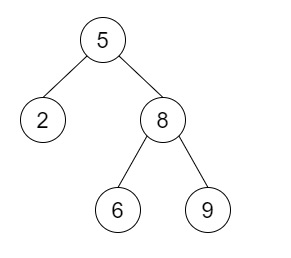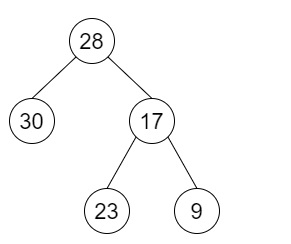# Convert BST to Greater Tree in C++

Suppose we have a Binary Search Tree, we have to convert it into a Greater Tree such that every key of the original BST is changed to the original key + sum of all keys greater than the original key in BST.

So, if the input is likethen the output will beTo solve this, we will follow these steps −

• Define a function revInorder(), this will take tree root and s,

• if root is null, then −

• return

• revInorder(right of root, s)

• s := s + val of root

• val of root := s

• revInorder(left of root, s)

• From the main method, do the following −

• if root is null, then −

• return null

• sum := 0

• revInorder(root, sum)

• return root

## Example

Let us see the following implementation to get a better understanding −

Live Demo

#include <bits/stdc++.h>
using namespace std;
class TreeNode{
public:
int val;
TreeNode *left, *right;
TreeNode(int data){
val = data;
left = NULL;
right = NULL;
}
};
void insert(TreeNode **root, int val){
queue<TreeNode*> q;
q.push(*root);
while(q.size()){
TreeNode *temp = q.front();
q.pop();
if(!temp->left){
if(val != NULL)
temp->left = new TreeNode(val);
else
temp->left = new TreeNode(0);
return;
}
else{
q.push(temp->left);
}
if(!temp->right){
if(val != NULL)
temp->right = new TreeNode(val);
else
temp->right = new TreeNode(0);
return;
}
else{
q.push(temp->right);
}
}
}
TreeNode *make_tree(vector<int> v){
TreeNode *root = new TreeNode(v);
for(int i = 1; i<v.size(); i++){
insert(&root, v[i]);
}
return root;
}
void tree_level_trav(TreeNode*root){
if (root == NULL) return;
cout << "[";
queue<TreeNode *> q;
TreeNode *curr;
q.push(root);
q.push(NULL);
while (q.size() > 1) {
curr = q.front();
q.pop();
if (curr == NULL){
q.push(NULL);
}
else {
if(curr->left)
q.push(curr->left);
if(curr->right)
q.push(curr->right);
if(curr == NULL || curr->val == 0){
cout << "null" << ", ";
}
else{
cout << curr->val << ", ";
}
}
}
cout << "]"<<endl;
}
class Solution {
public:
void revInorder(TreeNode *root,int &s){
if (root == NULL || root->val == 0)
return;
revInorder(root->right, s);
s += root->val;
root->val = s;
revInorder(root->left, s);
}
TreeNode* convertBST(TreeNode* root){
if (root == NULL || root->val == 0)
return NULL;
int sum = 0;
revInorder(root, sum);
return root;
}
};
main(){
Solution ob;
vector<int> v = {5,2,8,NULL,NULL,6,9};
TreeNode *root = make_tree(v);
tree_level_trav(ob.convertBST(root));
}

## Input

{5,2,8,NULL,NULL,6,9}

## Output

[28, 30, 17, null, null, 23, 9, ]